## Sunday, September 20, 2009

### Solving a 3x3 Magic Square

In my last post, From 1 Through 19, I challenged you to solve a 3x3 magic square. This is a small instance of the problem (the smallest solvable instance, a 1x1 magic square, is trivial), so it's possible to solve it using a brute force method, but the solution I'll give is a little more elegant than that.

As I wrote in my last post, a magic square is an arrangement of n2 distinct integers (from 1 to n2) in a square such that the n numbers in each row, each column, and in the two long diagonals all have the same sum.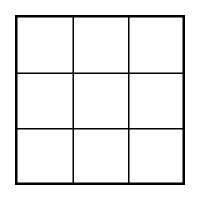The magic sum

The sum for each row, column, and diagonal is known as the magic sum for the magic square of that particular size. This sum is unique for each size magic square. Knowing the size of the square, there's an easy way to figure out the magic sum. For a 3x3 magic square, we know that each of the three rows add up to the magic sum, x. We also know that all the numbers from 1 through 9 must be used exactly once in all three rows. Combining these two facts we get

3x = 1 + 2 + 3 + 4 + 5 + 6 + 7 + 8 + 9
3x = 45
x = 15

So for our 3x3 magic square, the magic sum is 15. You can generalize the approach above to come up with the magic sum of any size magic square you want to solve.

The center square

The value that goes in the center square can be found using the same process we used in the From 1 Through 19 puzzle. Can the number 6 be placed in the center? No, because that would leave no place for the 9 (6 and 9 already add to 15). The numbers 7, 8, and 9 can be eliminated from the center for the same reason. What about placing the number 4 in the center? That won't do either, since then there would be no place we could put the 1 and still come up with a sum of 15. All the numbers 1 through 4 can be shown to be too small for the same reason. That leaves only the number 5 in the center square.

The rest of the numbers don't just fall into place, though, as they did in the From 1 Through 19 puzzle. Magic squares have additional constraints that weren't present in the earlier puzzle. We'll look at those constraints in the next sections.

9 in the corner?

To illustrate why the rest of the numbers don't fall easily into place after finding the center value, let's place the 9 in a corner and see what happens.It's plain to see that a number in a corner (in this case, the 9) must combine with six other numbers in three different ways (row, column, diagonal) to sum to 15. It's tempting to start plugging in numbers for the unknown values to see what works, but there's a problem with that approach.

Notice that in the image above there are only two blank squares that the 9 doesn't combine with to form a sum. We know that the 9 cannot be combined with any of 6, 7, or 8, since the sum would then be greater than our magic sum of 15. Since there's no way to force all three of 6, 7, and 8 into the two blank squares, we have no choice but to conclude that the 9 cannot go in a corner.

9 on the side

Once you eliminate the four corners, you have no choice but to place the 9 in one of the four side positions. It doesn't really matter which one you choose, since rotating the puzzle will give you the same solution. After placing the 9, the 1 also falls into place.Once again, it's tempting to start plugging in numbers to see what values work for the rest of the unknown squares, but there's a little bit more logic that can be applied before the remaining numbers fall into place. We already know that the numbers 6, 7, and 8 cannot be in the same row with the 9. That leaves only the 2, 3, and 4 as candidates for the squares I've labeled x and y in the diagram above. If we were to place the 3 in one of them, we would also need to place the 3 in the other to arrive at the magic sum of 15. Since we can only use each number once, this eliminates the 3 from being placed in the same row with the 9. That leaves only the 2 and 4.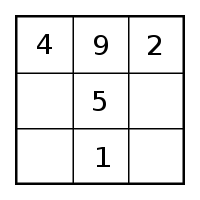Now there is finally enough information to begin filling in the remaining squares, starting with the two diagonals. One diagonal already contains the 4 and the 5, so the third value must be the 6. The other diagonal containing the 2 and the 5 can be completed only with the 8.The sum of 15 across the bottom row verifies that these values are correct. The only two values that we haven't used so far, 3 and 7, fall neatly into place for the final solution.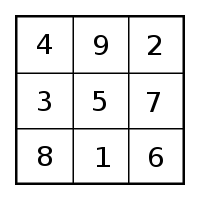If this article doesn't contain everything you ever wanted to know about magic squares (and I understand that some of you probably never even wanted to know this much), then check out the book Before Sudoku: The World of Magic Squares. It contains the history, several variations, and even a few practical applications for this fascinating puzzle.

## Sunday, September 13, 2009

### From 1 Through 19

The Problem

I found the following puzzle in a book called the Moscow Puzzles by Boris A. Kordemsky. (It's problem number 34, which you can also find at Google books.)

Write the numbers from 1 through 19 in the circles so that the numbers in every 3 circles on a straight line total 30.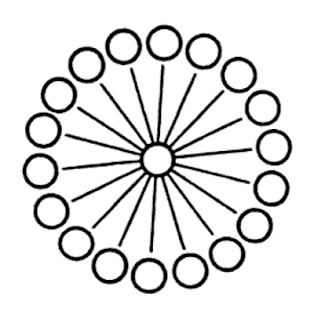Take a minute to try and solve it on your own before reading on. (It's not that hard, so it really should take just a minute or so.)

The Solution

The key to this puzzle is figuring out which number goes in the center. It's easy to show what numbers can't be in the center. The 1 can't be in the center because, although there are a few combinations you can come up with that add up to 30, there aren't enough with 1 in the center position. You could have the combinations

19, 1, 10
18, 1, 11
17, 1, 12
...

But you'll soon be left with numbers too small to form a group that sums to 30. For example, what number do you place across from the 2?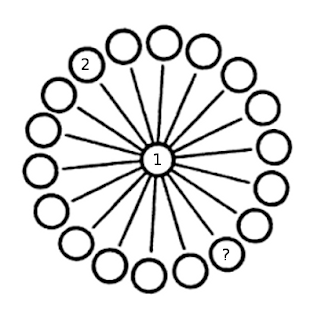There aren't any numbers large enough to fill the third spot in this combination. You can try placing each of the numbers from 1 through 9 in the center and find the same shortcoming.

Similarly, the number 19 is too large to go in the center. There's no number you can place opposite the 18. In fact the sum of 18 and 19 is already too large, even without a number in the opposite circle.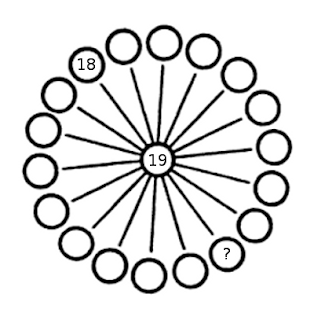Through trial and error, you can find that all of the numbers from 11 through 19 are too large.

That leaves only the number 10 to occupy center position. After that, all of the other numbers fall into place.

A Challenge

A magic square is an arrangement of n2 distinct integers (from 1 to n2) in a square such that the n numbers in each row, each column, and in the two long diagonals all have the same sum.

Using the principles from the solution to the From 1 Through 19 puzzle above, can you construct a 3 x 3 magic square?I'll give you the additional piece of information that each row, column, and diagonal must add to the sum of 15 (to save you the trouble of looking it up on Wikipedia, which would be cheating.)

I'll have the complete solution in an upcoming post.

## Wednesday, September 2, 2009

### Getting a Fair Toss From a Biased Coin

A paper out of Stanford and UCSC, Dynamical Bias in the Coin Toss (PDF, 5MB), reveals a small but significant bias in the process of flipping a coin.

James Devlin of Coding the Wheel provides the following summary:
1. If the coin is tossed and caught, it has about a 51% chance of landing on the same face it was launched. (If it starts out as heads, there's a 51% chance it will end as heads).
2. If the coin is spun, rather than tossed, it can have a much-larger-than-50% chance of ending with the heavier side down. Spun coins can exhibit "huge bias" (some spun coins will fall tails-up 80% of the time).
3. If the coin is tossed and allowed to clatter to the floor, this probably adds randomness.
4. If the coin is tossed and allowed to clatter to the floor where it spins, as will sometimes happen, the above spinning bias probably comes into play.
5. A coin will land on its edge around 1 in 6000 throws, creating a flipistic singularity.
6. The same initial coin-flipping conditions produce the same coin flip result. That is, there's a certain amount of determinism to the coin flip.
7. A more robust coin toss (more revolutions) decreases the bias.

The article goes on to suggest a coin-flipping strategy that tries to take advantage of the small bias, but what about getting a completely fair toss from a biased coin?

It turns out to be fairly easy. Just follow these steps:
1. Flip the coin twice.
2. If both tosses are the same (heads-heads or tails-tails), repeat step 1.
3. If the tosses come up heads-tails, count the toss as heads. If the tosses come up tails-heads, count it as tails.
To see why this method makes even a biased coin fair, let's pretend we have a weighted coin that comes up heads 60% of the time. If you toss it twice and throw out the result when both tosses are the same, you're left with two possible outcomes. The probabilities of the two remaining outcomes are the same.

P(HT) = P(TH)
P(H) * P(T) = P(T) * P(H)
0.6 * 0.4 = 0.6 * 0.4 = 0.24

Since both outcomes have exactly the same probability, the bias is removed. This method will work no matter how biased the coin you use, as long as there's some possibility of it coming up either heads or tails (so no two-headed coins allowed).

Note: This method for simulating a fair coin using a biased coin was introduced by John von Neumann in Various techniques used in connection with random digits (1951).